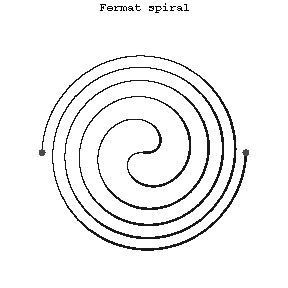Fermat spiral

$$\rho=a\sqrt\phi.$$
To each value of $\phi$ correspond two values of $\rho$ — a positive and a negative one. The Fermat spiral is centrally symmetric relative to the pole, which is a point of inflection. It belongs to the class of so-called algebraic spirals.